Next: -ray Radiation from the Up: ph_rad Previous: Transportation of the VHE

The electromagnetic (EM) process are always related to photons because they are the particles to carry energy and momentum in electromagnetic interactions. Therefore, some of the EM reactions would GENERATE photons, such as pair annihilation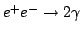, the inverse process of the pair creation. Electrons are also producing photons by interacting with magnetic field, which is called synchrotron radiation, the representative radiation process that can be described in the standard context of the classical electromagnetic theory.

Now let us derive the radiation intensity, i.e. the radiated energy per unit time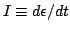. First we expand the treatment of the dipole radiation given by Eq. 8 to the super-relativistic condition that particles are moving almost at speed of light. The dipole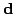is related to acceleration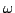by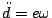and Eq. 8 gives the total radiation intensity as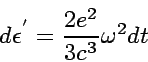(37)

The radiated 4-momentum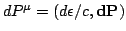should be equivalent to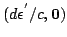when electron is at rest. We find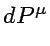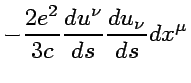(38)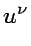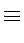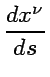since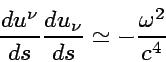(39)

in limit of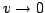. The time component (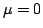) of Eq. 39 gives the total radiation intensity, which reads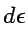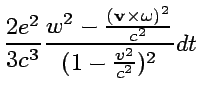(40)

The equation of motion in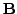and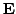is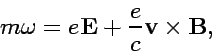(41)

then we finally obtain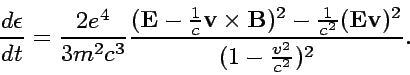(42)

In the uniformfield, an electron's movement is characterized by the synchrotron frequency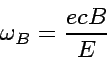(43)

whereis energy of a charged particle. Then Eq. 42 leads to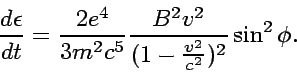(44)

Here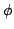is a pitch angle between electron momentum andfield. In ultra-relativistic case of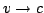, we obtain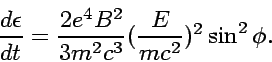(45)

Averaging the pitch angle and using the Thompson scattering cross section 12 gives the final expression: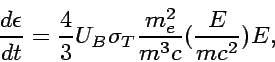(46)

where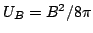is the energy density of magnetic field. When a particle moving in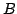is an electron,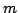is set to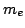in the above equation.

Energies of the radiated photons are narrowly distributed around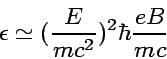(47)

SubsectionsNext: -ray Radiation from the Up: ph_rad Previous: Transportation of the VHE
Shigeru Yoshida
2002-07-18